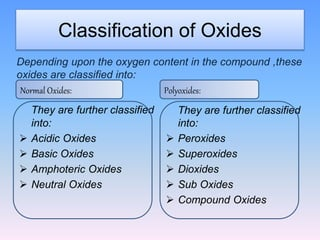# Classification of oxides

• A binary compound of oxygen with another element is known as an oxide.
• There are various types of oxides. They are as follows:

A) Basic oxides:

• It is formed with alkali metals, alkaline earth metals (except Be) and transition metals generally.
• These oxides give bases with water.
• These oxides react with acids to form salt and water.
• For examples: Na2O, K2O, CaO, FeO, Pbo, etc.

Na2O + H2O → 2NaOH          Na2O + 2HCl → 2NaCl + H2O

B) Acidic oxides:

• Non-metals and transition metals in higher oxidation state (CO2, SO3, N2O5, V2O5, Mn2O7, CrO3 ) form acidic oxides.
• These combine with water to give acid.
• These oxides react with bases to give salt and water.

CO2 + H2O  →    H2CO3           SO2 + 2NaOH  →  Na2SO3 + H2O

C) Amphoteric oxides:

• These are formed by amphoteric oxides.
• These react with both acids and bases.
• Examples: Al2O3, BeO, SnO, ZnO, etc.

Al2O3 + 6HCl →2AlCl3 + 3H2O

D) Neutral oxides:

• These neither give acids nor bases with water.
• For example: CO, H2O, N2O, NO, etc.

E) Normal oxides:

• In these oxides, oxygen content is as much as permitted by the normal valency of its metal.
• For examples: H2O, Al2O3, etc.
• These contain only M-O bond.Image source: slideplayer

F) Poly- oxides:

• These oxides contain more oxygen than permitted by the normal valency of its metal.
• These are of various types. They are:

i)They give H2O2 with water or acid.

ii)In these oxides oxidation state of oxygen is -1.

iii)For example: BaO2, Na2O2, etc.

b) Super-oxides:

i)Oxidation state of oxygen is -1/2.

ii)They contain O22-.

iii)For examples: KO2, RbO2 and CsO2.

c) Dioxides:

i) Poly-oxides with higher percentage of oxygen.

ii)They do not yield H2O2 with water or acid.

iii)For example: MnO2, PbO2, etc.

G) Sub- oxides:

• Oxides containing less oxygen than permitted by normal valency of its metal are sub-oxides.
• For examples: N2O, C3O2 (O=C=C=C=O).

H) Mixed oxides:

• These are the oxides made up of two similar oxides.
• For examples: red lead Pb3O4 (2PbO + PbO2) and ferroso-feric oxide/ loadstone Fe3O4 (FeO + Fe2O3)

References: